# Pythagoras

Determines the hypotenuse or leg of a right triangle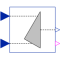# Information

This information is part of the Modelica Standard Library maintained by the Modelica Association.

This block determines the hypotenuse `y = sqrt(u1^2 + u2^2)` if the boolean parameter `u1IsHyotenuse = false`. In this case the two inputs `u1` and `u2` are interpreted as the legs of a right triangle and the boolean output `valid` is always equal to `true`.

If `u1IsHyotenuse = true`, input `u1` is interpreted as hypotenuse and `u2` is one of the two legs of a right triangle. Then, the other of the two legs of the right triangle is the output, determined by `y = sqrt(u1^2 - u2^2)`, if `u1^2 - u2^2 ≥ 0`; in this case the boolean output `valid` is equal to `true`. In case of `u1^2 - u2^2 < 0`, the output `y = 0` and `valid` is set to `false`.

# Parameters (1)

u1IsHypotenuse Value: false Type: Boolean Description: If true, u1 is the hypotenuse and y is one leg

# Connectors (4)

u1 u2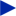Type: RealInput Description: Connector of Real input signal 1Type: RealInput Description: Connector of Real input signal 2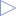Type: RealOutput Description: Connector of Real output signal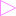Type: BooleanOutput Description: Is true, if y is a valid result

# Used in Components (1)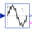TotalHarmonicDistortion Modelica.Blocks.Math Output the total harmonic distortion (THD)